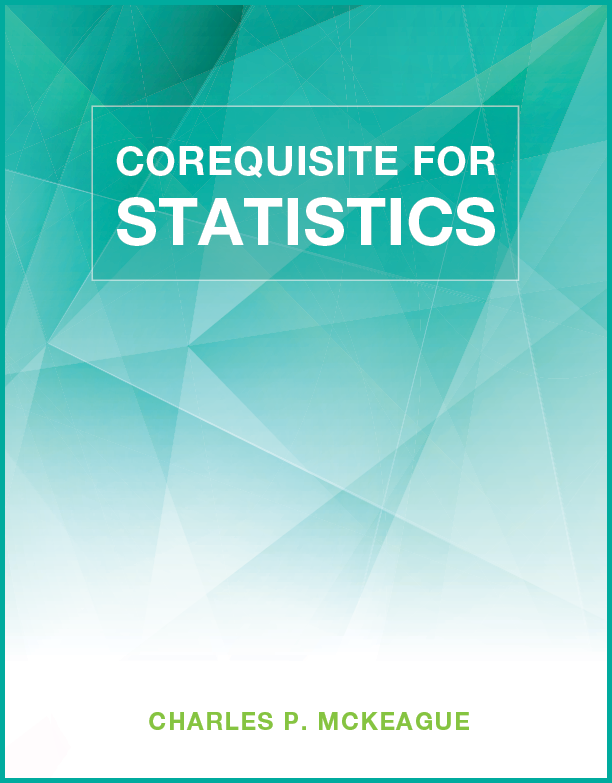## Corequisite for Statistics

### Author: Charles P. McKeagueVideo player setup failed. Please make sure you have JavaScript enabled in your browser settings. If necessary, try another web browser that supports HTML5 video.

Lessons:

Lesson 1: Definitions, Order of Operations, Simplifying Expressions, and Sets
Lesson 2: Negative Numbers, Opposites, Reciprocals, and Square Roots
Lesson 3: Arithmetic with Negative Numbers
Lesson 4: Mean, Median, Mode, Standard Deviation, and Summation Notation
Lesson 5: Solving Equations and Formulas
Lesson 6: Percent, Decimals, and Fractions
Lesson 7: Inequalities
Lesson 8: Ordered Pairs and Graphing
Lesson 9: Slope and Rates of Change
Lesson 10: Equations of Lines
Lesson 11: Coefficient of Correlation and Linear Regression
Lesson 12: Fundamental Counting Principle
Lesson 13: Permutations and Combinations
Lesson 14: Introduction to Probability